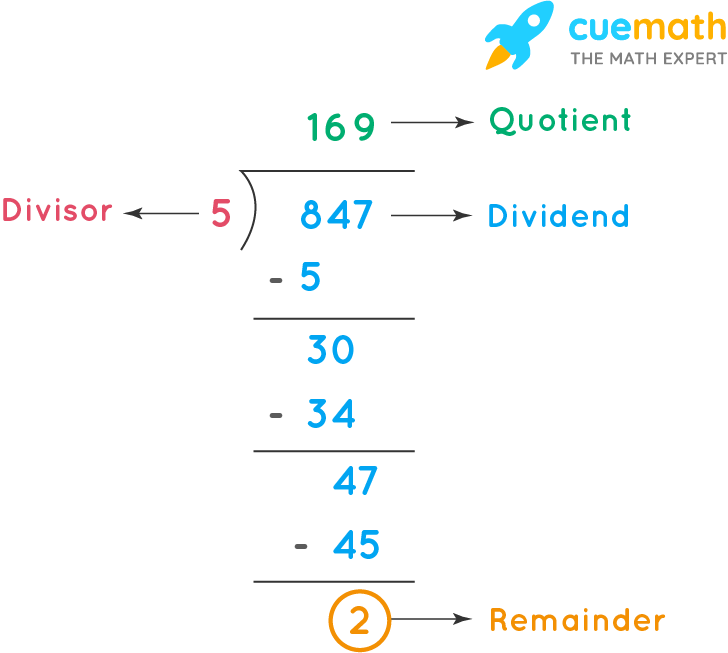# Division Calculator With Remainders Calculator

## What is Division With Remainders Calculator?

'Cuemath's Division With Remainders Calculator' is an online tool that helps to find the remainder. Cuemath's online division with remainders calculator helps you to find the remainder within a few seconds.

## How to Use Division With Remainders Calculator?

• Step 1: Enter the dividend and the divisor in the input boxes.
• Step 2: Click on the "Divide" button to find the remainder.
• Step 3: Click on "Reset" to clear the field and enter the new values.

## What Is Division With Remainders Calculator?

The Remainder is defined as the number or value that is left when the dividend is not completely divisible by the divisor.

Division (÷) is an arithmetic operation in which we distribute a group of things into equal parts.

The terms used in the division are as follows:

• Dividend - is the number that is divided
• Divisor - is the number that divides the dividend.
• Quotient - is the result obtained.
• The remainder - is the number or value that is left when the dividend is not completely divisible by the divisor

The formula used for the division is Dividend = Divisor x Quotient + Remainder

Remainder = Dividend - (Divisor x Quotient)

### Solved Example:

Find the remainder when 845 is divided by 5

### Solution:

To find: Remainder

Given: Dividend = 845 and Divisor = 5

By long division method:Therefore, the remainder is 2.

Similarly, you can try the calculator to find the remainder for the following:

• When 7085 is divided by 8
• When 8021 is divided by 9